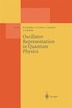# Oscillator Representation in Quantum Physics

## byM. Dineykhan, G.V. Efimov, G. Ganbold

### Paperback | April 18, 2014\$127.11 online
\$142.95 list price save 11%
Earn 636 plum® points
Prices and offers may vary in store
Special OfferSee details
Quantity:

In stock online

Ships free on orders over \$35

Not available in stores

The investigation ofmost problems of quantum physics leads to the solution of the Schrodinger equation with an appropriate interaction Hamiltonian or potential. However, the exact solutions are known for rather a restricted set of potentials, so that the standard eternal problem that faces us is to find the best effective approximation to the exact solution of the Schrodinger equation under consideration. In the most general form, this problem can be formulated as follows. Let a total Hamiltonian H describing a relativistic (quantum field theory) or a nonrelativistic (quantum mechanics) system be given. Our problem is to solve the Schrodinger equation Hlft = Enlftn, n i. e. , to find the energy spectrum {En} and the proper wave functions {lft } n including the'ground state or vacuum lft = 10). The main idea of any ap­ o proximation technique is to find a decomposition in such a way that Ha describes our physical system in the "closest to H" manner, and the Schrodinger equation HolJt. (O) = E(O)lJt. (O) n n n can be solved exactly. The interaction Hamiltonian HI is supposed to give small corrections to the zero approximation which can be calculated. In this book, we shall consider the problem of a strong coupling regime in quantum field theory, calculations ofpath or functional integrals over the Gaussian measure and spectral problems in quantum mechanics. Let us con­ sider these problems briefly.
The Phase Structure of Quantum Field Systems.- Formulation of the Method.- The Phase Structure of the (?2)2 Field Theory in R1+1.- The Phase Structure of the Three-Dimensional ?4 Theory.- The Four-Dimensional ?4 Theory.- The ?4 Theory at Finite Temperatures.- The Two-Dimensional Yukawa Theory.- The Gaussian Equivalent Representation of...